# RD Sharma Solutions for Class 8 Maths Chapter 9 Linear Equation in One Variable Exercise 9.1

In Exercise 9.1 of Chapter 9, we shall deal with equations involving rational numbers as the coefficients and their solutions can also be rational numbers. We shall also solve equations having variable terms on one side and numbers on the other side. For 8th grade, RD Sharma Solutions is the best reference material for students as it is based on CBSE board pattern. Tutors at BYJU’S have developed the solutions for RD Sharma Class 8 Maths in a simple and easily understandable language to help students secure high marks in their exams. Download the pdf from the links given below.

## Download the pdf of RD Sharma Solutions for Class 8 Maths Exercise 9.1 Chapter 9 Linear Equation in One Variable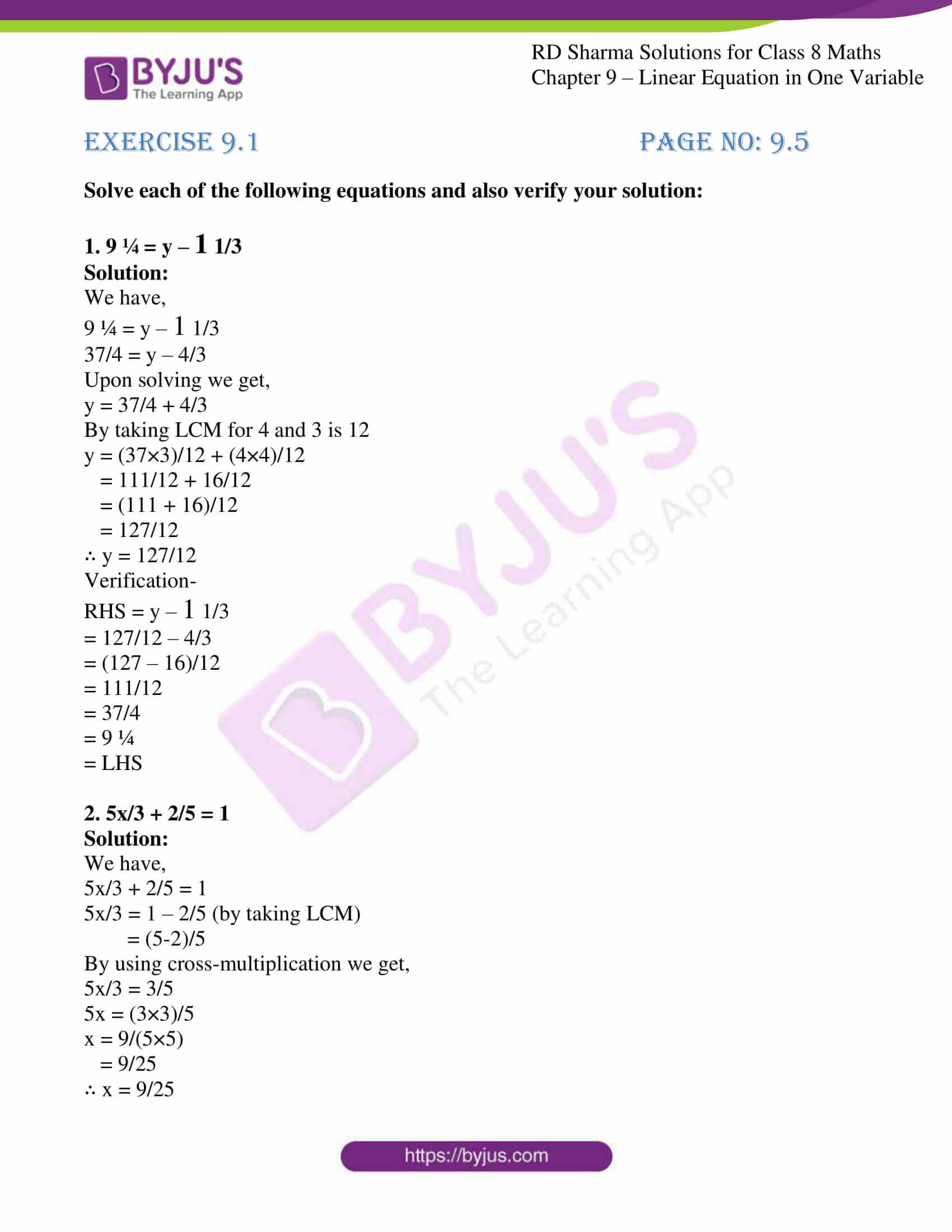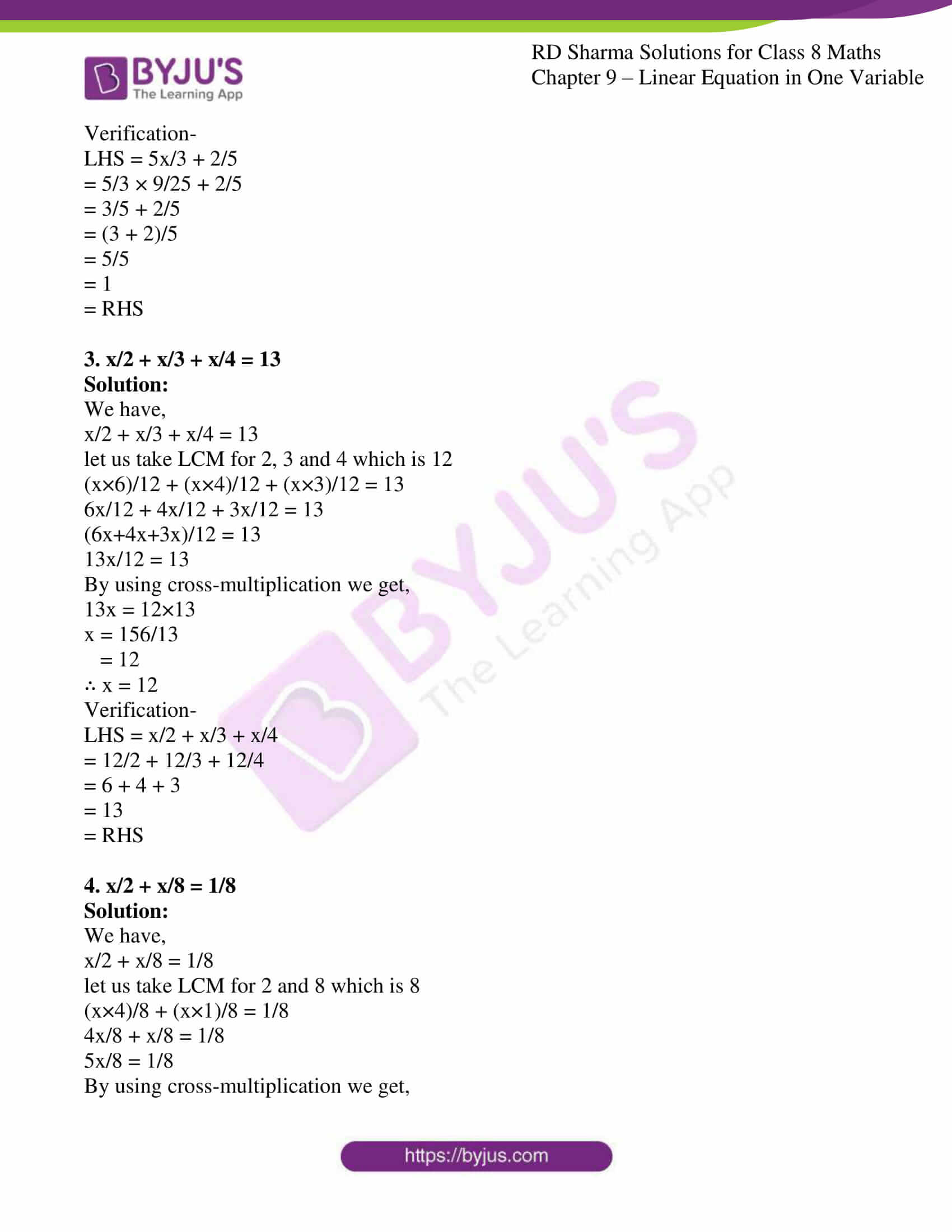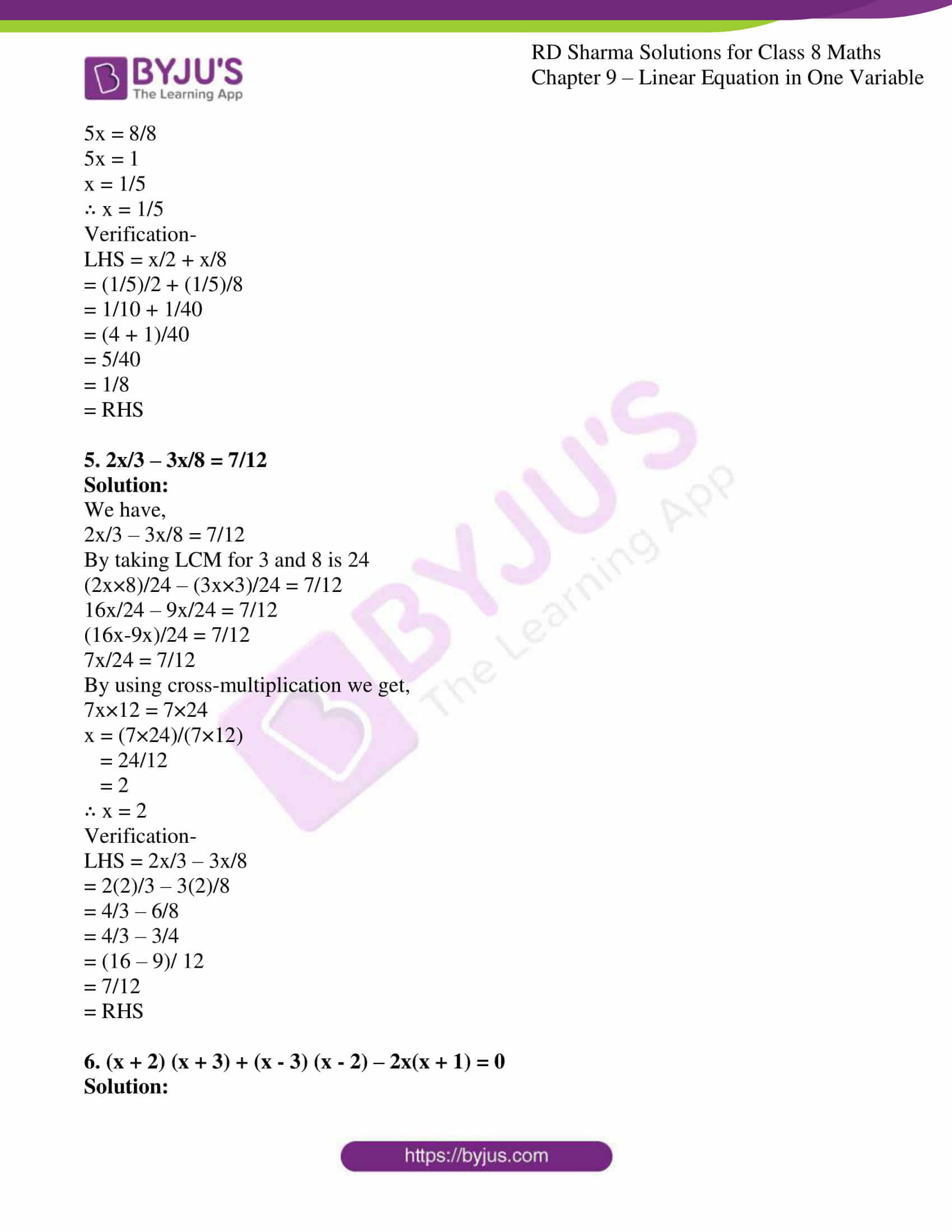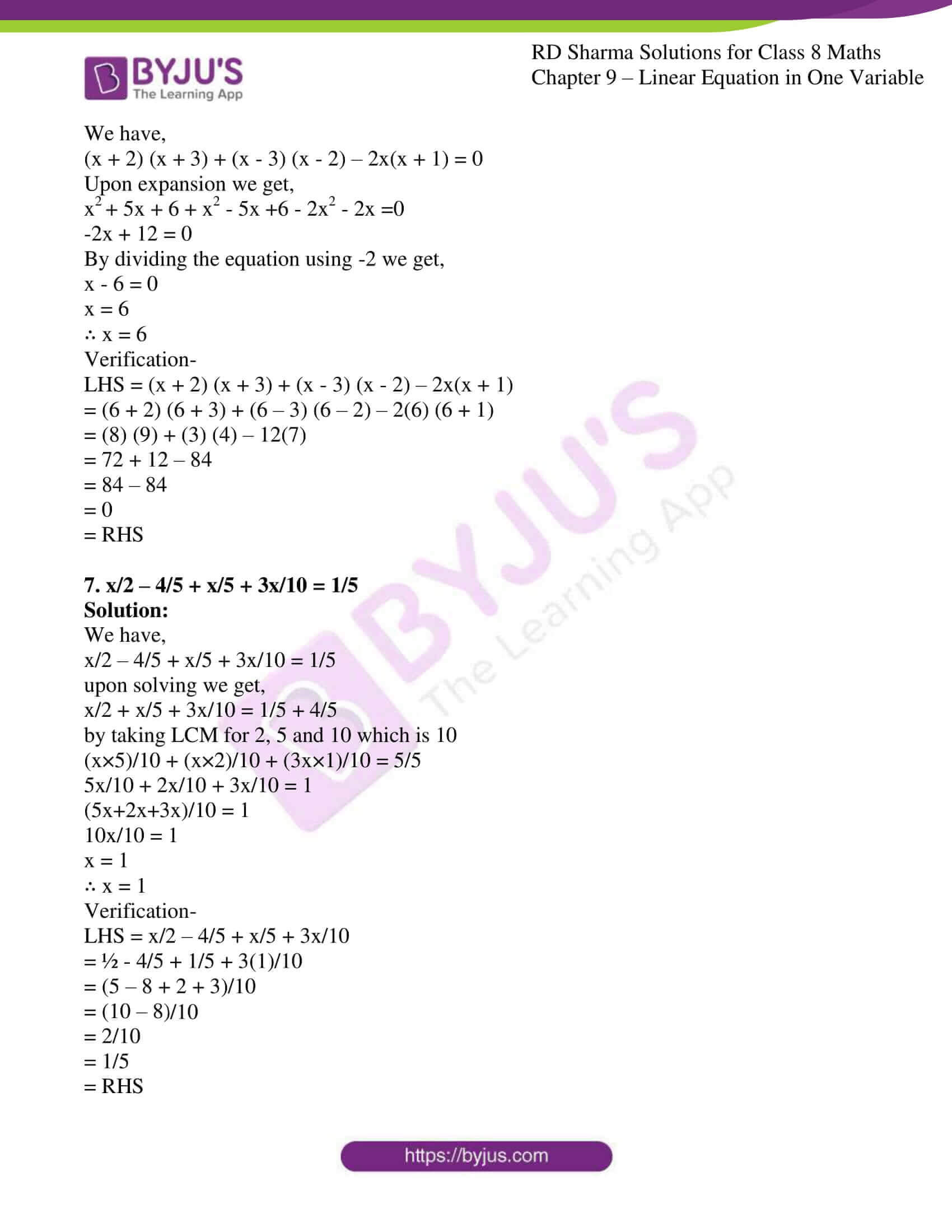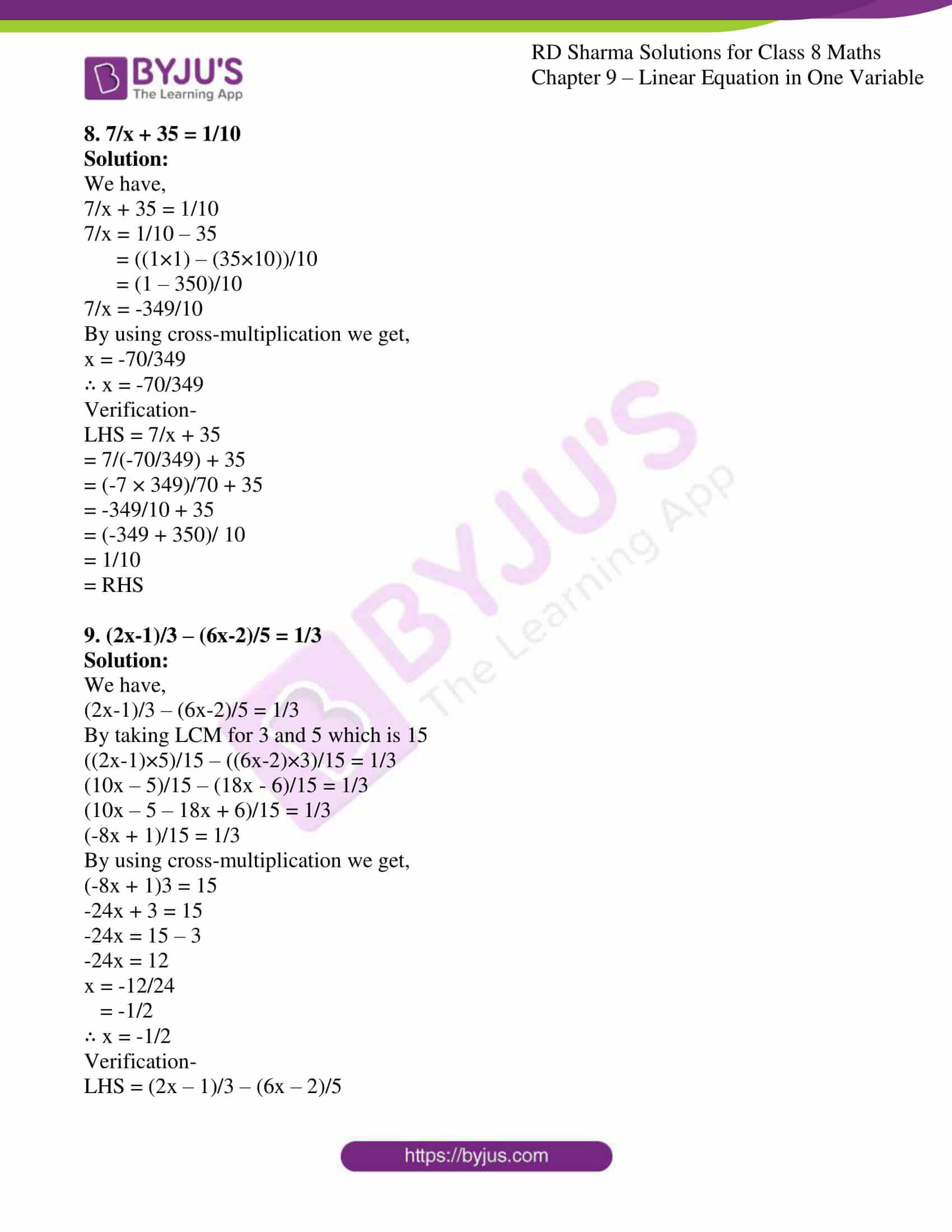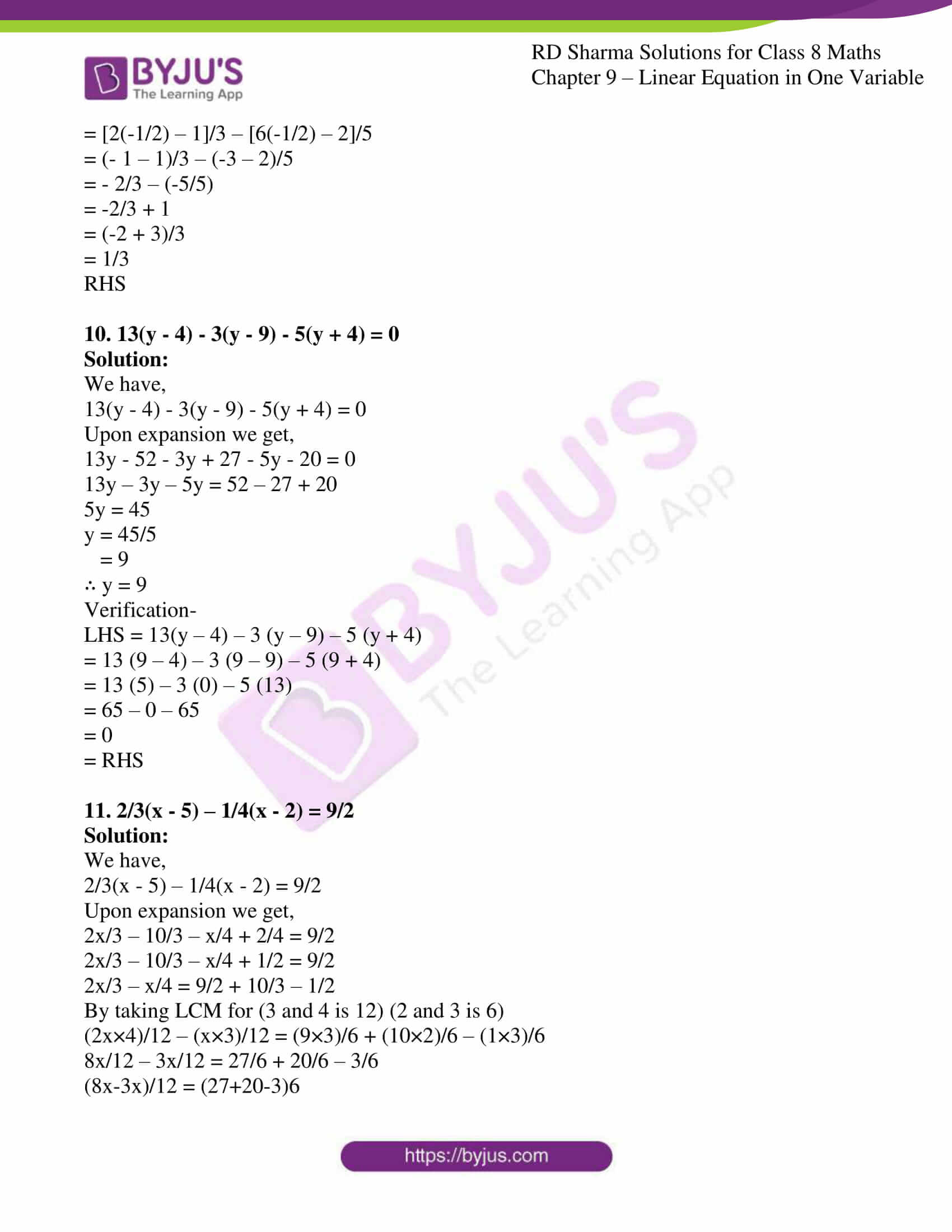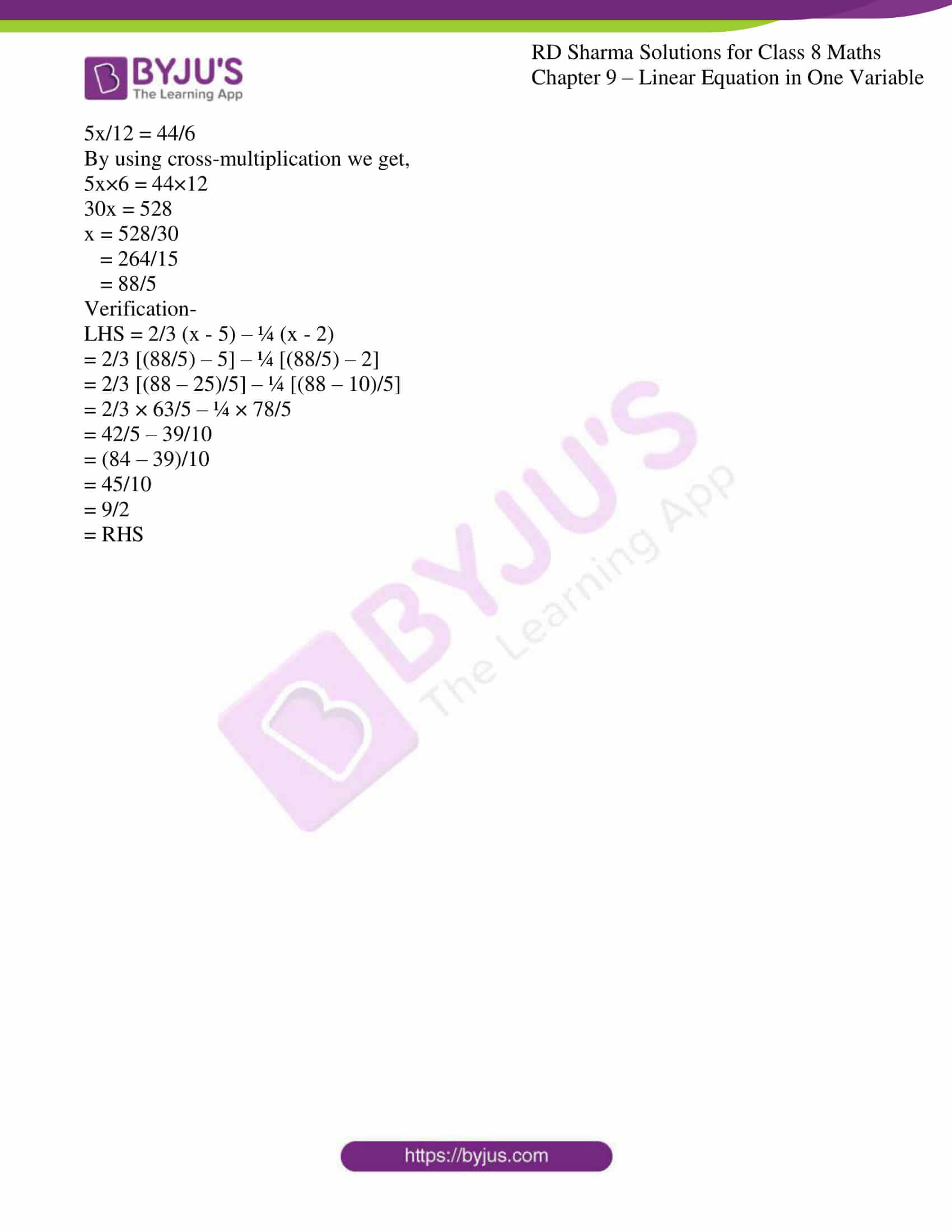### Access Answers to RD Sharma Solutions for Class 8 Maths Exercise 9.1 Chapter 9 Linear Equation in One Variable

#### EXERCISE 9.1 PAGE NO: 9.5

Solve each of the following equations and also verify your solution:

1. 9 ¼ = y – 1 1/3

Solution:

We have,

9 ¼ = y – 1 1/3

37/4 = y – 4/3

Upon solving we get,

y = 37/4 + 4/3

By taking LCM for 4 and 3 is 12

y = (37×3)/12 + (4×4)/12

= 111/12 + 16/12

= (111 + 16)/12

= 127/12

∴ y = 127/12

Verification-

RHS = y – 1 1/3

= 127/12 – 4/3

= (127 – 16)/12

= 111/12

= 37/4

= 9 ¼

= LHS

2. 5x/3 + 2/5 = 1

Solution:

We have,

5x/3 + 2/5 = 1

5x/3 = 1 – 2/5 (by taking LCM)

= (5-2)/5

By using cross-multiplication we get,

5x/3 = 3/5

5x = (3×3)/5

x = 9/(5×5)

= 9/25

∴ x = 9/25

Verification-

LHS = 5x/3 + 2/5

= 5/3 × 9/25 + 2/5

= 3/5 + 2/5

= (3 + 2)/5

= 5/5

= 1

= RHS

3. x/2 + x/3 + x/4 = 13

Solution:

We have,

x/2 + x/3 + x/4 = 13

let us take LCM for 2, 3 and 4 which is 12

(x×6)/12 + (x×4)/12 + (x×3)/12 = 13

6x/12 + 4x/12 + 3x/12 = 13

(6x+4x+3x)/12 = 13

13x/12 = 13

By using cross-multiplication we get,

13x = 12×13

x = 156/13

= 12

∴ x = 12

Verification-

LHS = x/2 + x/3 + x/4

= 12/2 + 12/3 + 12/4

= 6 + 4 + 3

= 13

= RHS

4. x/2 + x/8 = 1/8

Solution:

We have,

x/2 + x/8 = 1/8

let us take LCM for 2 and 8 which is 8

(x×4)/8 + (x×1)/8 = 1/8

4x/8 + x/8 = 1/8

5x/8 = 1/8

By using cross-multiplication we get,

5x = 8/8

5x = 1

x = 1/5

∴ x = 1/5

Verification-

LHS = x/2 + x/8

= (1/5)/2 + (1/5)/8

= 1/10 + 1/40

= (4 + 1)/40

= 5/40

= 1/8

= RHS

5. 2x/3 – 3x/8 = 7/12

Solution:

We have,

2x/3 – 3x/8 = 7/12

By taking LCM for 3 and 8 is 24

(2x×8)/24 – (3x×3)/24 = 7/12

16x/24 – 9x/24 = 7/12

(16x-9x)/24 = 7/12

7x/24 = 7/12

By using cross-multiplication we get,

7x×12 = 7×24

x = (7×24)/(7×12)

= 24/12

= 2

∴ x = 2

Verification-

LHS = 2x/3 – 3x/8

= 2(2)/3 – 3(2)/8

= 4/3 – 6/8

= 4/3 – 3/4

= (16 – 9)/ 12

= 7/12

= RHS

6. (x + 2) (x + 3) + (x – 3) (x – 2) – 2x(x + 1) = 0

Solution:

We have,

(x + 2) (x + 3) + (x – 3) (x – 2) – 2x(x + 1) = 0

Upon expansion we get,

x+ 5x + 6 + x2 – 5x +6 – 2x2 – 2x =0

-2x + 12 = 0

By dividing the equation using -2 we get,

x – 6 = 0

x = 6

∴ x = 6

Verification-

LHS = (x + 2) (x + 3) + (x – 3) (x – 2) – 2x(x + 1)

= (6 + 2) (6 + 3) + (6 – 3) (6 – 2) – 2(6) (6 + 1)

= (8) (9) + (3) (4) – 12(7)

= 72 + 12 – 84

= 84 – 84

= 0

= RHS

7. x/2 – 4/5 + x/5 + 3x/10 = 1/5

Solution:

We have,

x/2 – 4/5 + x/5 + 3x/10 = 1/5

upon solving we get,

x/2 + x/5 + 3x/10 = 1/5 + 4/5

by taking LCM for 2, 5 and 10 which is 10

(x×5)/10 + (x×2)/10 + (3x×1)/10 = 5/5

5x/10 + 2x/10 + 3x/10 = 1

(5x+2x+3x)/10 = 1

10x/10 = 1

x = 1

∴ x = 1

Verification-

LHS = x/2 – 4/5 + x/5 + 3x/10

= ½ – 4/5 + 1/5 + 3(1)/10

= (5 – 8 + 2 + 3)/10

= (10 – 8)/10

= 2/10

= 1/5

= RHS

8. 7/x + 35 = 1/10

Solution:

We have,

7/x + 35 = 1/10

7/x = 1/10 – 35

= ((1×1) – (35×10))/10

= (1 – 350)/10

7/x = -349/10

By using cross-multiplication we get,

x = -70/349

∴ x = -70/349

Verification-

LHS = 7/x + 35

= 7/(-70/349) + 35

= (-7 × 349)/70 + 35

= -349/10 + 35

= (-349 + 350)/ 10

= 1/10

= RHS

9. (2x-1)/3 – (6x-2)/5 = 1/3

Solution:

We have,

(2x-1)/3 – (6x-2)/5 = 1/3

By taking LCM for 3 and 5 which is 15

((2x-1)×5)/15 – ((6x-2)×3)/15 = 1/3

(10x – 5)/15 – (18x – 6)/15 = 1/3

(10x – 5 – 18x + 6)/15 = 1/3

(-8x + 1)/15 = 1/3

By using cross-multiplication we get,

(-8x + 1)3 = 15

-24x + 3 = 15

-24x = 15 – 3

-24x = 12

x = -12/24

= -1/2

∴ x = -1/2

Verification-

LHS = (2x – 1)/3 – (6x – 2)/5

= [2(-1/2) – 1]/3 – [6(-1/2) – 2]/5

= (- 1 – 1)/3 – (-3 – 2)/5

= – 2/3 – (-5/5)

= -2/3 + 1

= (-2 + 3)/3

= 1/3

RHS

10. 13(y – 4) – 3(y – 9) – 5(y + 4) = 0

Solution:

We have,

13(y – 4) – 3(y – 9) – 5(y + 4) = 0

Upon expansion we get,

13y – 52 – 3y + 27 – 5y – 20 = 0

13y – 3y – 5y = 52 – 27 + 20

5y = 45

y = 45/5

= 9

∴ y = 9

Verification-

LHS = 13(y – 4) – 3 (y – 9) – 5 (y + 4)

= 13 (9 – 4) – 3 (9 – 9) – 5 (9 + 4)

= 13 (5) – 3 (0) – 5 (13)

= 65 – 0 – 65

= 0

= RHS

11. 2/3(x – 5) – 1/4(x – 2) = 9/2

Solution:

We have,

2/3(x – 5) – 1/4(x – 2) = 9/2

Upon expansion we get,

2x/3 – 10/3 – x/4 + 2/4 = 9/2

2x/3 – 10/3 – x/4 + 1/2 = 9/2

2x/3 – x/4 = 9/2 + 10/3 – 1/2

By taking LCM for (3 and 4 is 12) (2 and 3 is 6)

(2x×4)/12 – (x×3)/12 = (9×3)/6 + (10×2)/6 – (1×3)/6

8x/12 – 3x/12 = 27/6 + 20/6 – 3/6

(8x-3x)/12 = (27+20-3)6

5x/12 = 44/6

By using cross-multiplication we get,

5x×6 = 44×12

30x = 528

x = 528/30

= 264/15

= 88/5

Verification-

LHS = 2/3 (x – 5) – ¼ (x – 2)

= 2/3 [(88/5) – 5] – ¼ [(88/5) – 2]

= 2/3 [(88 – 25)/5] – ¼ [(88 – 10)/5]

= 2/3 × 63/5 – ¼ × 78/5

= 42/5 – 39/10

= (84 – 39)/10

= 45/10

= 9/2

= RHS